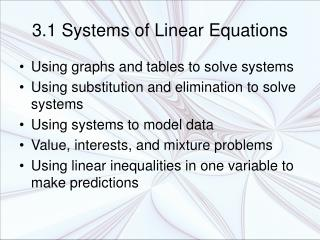DownloadDownload Presentation3.1 Systems of Linear Equations

# 3.1 Systems of Linear Equations

Télécharger la présentation## 3.1 Systems of Linear Equations

- - - - - - - - - - - - - - - - - - - - - - - - - - - E N D - - - - - - - - - - - - - - - - - - - - - - - - - - -
##### Presentation Transcript

1. 3.1 Systems of Linear Equations • Using graphs and tables to solve systems • Using substitution and elimination to solve systems • Using systems to model data • Value, interests, and mixture problems • Using linear inequalities in one variable to make predictions

2. Using Two Models to Make a Prediction • When will the life expectancy of men and women be equal? • L = W(t) = 0.144t + 77.47 • L = M(t) = 0.204t + 69.90 Equal at approximately 87 years old in 2064. 100 Years of Life 80 (84.11, 87.06) 60 40 60 80 100 120 20 Years since 1980

3. System of Linear Equations in Two Variables (Linear System) • Two or more linear equations containing two variables y = 3x + 3 y = -x – 5

4. Solution of a System • An ordered pair (a,b) is a solution of a linear system if it satisfies both equations. • The solution sets of a system is the set of all solutions for that system. • To solve a system is to find its solution set. • The solution set can be found by finding the intersection of the graphs of the two equations.

5. Find the Ordered Pairs that Satisfy Both Equations • Graph both equations on the same coordinate plane • y = 3x + 3 • y = -x – 5 • Verify • (-3) = 3(-2) + 3 • -3 = -6 + 3 • -3 = -3 • (-3) = -(-2) – 5 • -3 = 2 – 5 • -3 = -3 • Only one point satisfies both equations • (-2,-3) is the solution set of the system Solutions for y = 3x + 3 Solutions for y = -x – 5 Solution for both (-2,-3)

6. Example • ¾x + ⅜y = ⅞ • y = 3x – 5 • Solve first equation for y • ¾x + ⅜y = ⅞ • 8(¾x + ⅜y) = 8(⅞) • 24x + 24y = 56 4 8 8 • 6x -6x + 3y = 7 – 6x • 3y = -6x + 7 3 3 3 • y = -2x + 7/3

7. y = 3x – 5 -.6 = 3(1.45) – 5 -.6 = 4.35 – 5 -.6 ≈ -.65 y = -2x + 7/3 -.6 = -2(1.45) + 7/3 -.6 = -2.9 + 7/3 -.6 ≈ -.57 (1.45,-.6)

8. Inconsistent System • A linear system whose solution set is empty • Example…Parallel lines never intersect • no ordered pairs satisfy both systems

9. Dependent System • A linear system that has an infinite number of solutions • Example….Two equations of the same line • All solutions satisfy both lines y = 2x – 2 -2x + y = -2 • -2x +2x + y = -2 +2x • y = 2x – 2

10. One Solution System • There is exactly one ordered pair that satisfies the linear system • Example…Two lines that intersect in only one point

11. Solving Systems with Tables • Since (2,2) is a solution to both equations, it is a solution of the linear system.